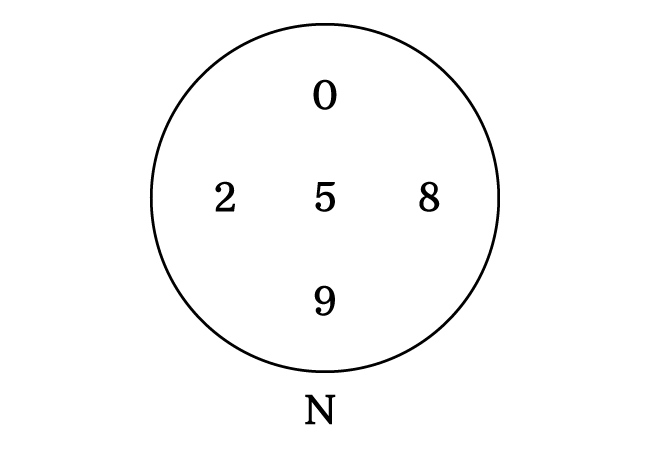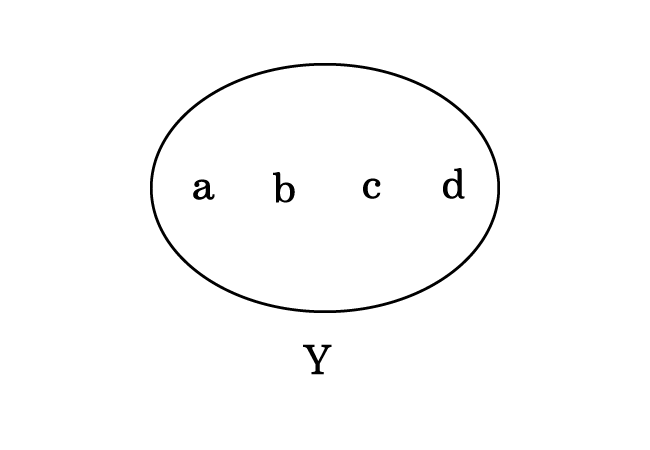# Belongs to

A symbol that expresses the phrase “to be a member of” in the set theory is called the belongs to symbol.

## IntroductionIn the set theory, the elements (or members) are collected on the basis of one or more common properties to form a set. So, each element is a member of that set. Hence, it is simply expressed as the element belongs to the set.

An Italian mathematician, Giuseppe Peano used a Greek letter lunate epsilon ($∈$) for expressing the phrase “belongs to” symbolically in set theory. It helps us to express the relationship between an element and its set in mathematical form.

### Usage

Let’s learn how to use the symbol epsilon in set theory from two understandable examples.

#### Basic example

The numbers $0$, $2$, $5$, $8$ and $9$ are collected to form a set $N$ in this example.1. The number $0$ is a member of set $N$. Hence, it is expressed as $0 \,∈\, N$.
2. The number $2$ is an element of set $N$. So, it is written as $2 \,∈\, N$.
3. The number $5$ belongs to set $N$. Therefore, the relationship between them is written as $5 \,∈\, N$.
4. The number $8$ is in set $N$ and it is expressed as $8 \,∈\, N$.
5. The number $9$ lies in set $N$ and it is written as $9 \,∈\, N$.

Thus, we use the epsilon symbol in set theory to express the relationship between an element and a set in mathematical form.The lowercase letters $a$, $b$, $c$ and $d$ are collected to form a set $Y$ in this example.

1. The letter $a$ is a member of set $Y$. Hence, it is expressed as $a \,∈\, Y$.
2. The letter $b$ is an element of set $Y$. So, it is written as $b \,∈\, Y$.
3. The letter $c$ belongs to set $Y$. Therefore, the relationship between them is written as $c \,∈\, Y$.
4. The letter $d$ is in set $Y$ and it is expressed as $d \,∈\, Y$.
Latest Math Topics
Jun 26, 2023
Jun 23, 2023

###### Math Questions

The math problems with solutions to learn how to solve a problem.

Learn solutions

Practice now

###### Math Videos

The math videos tutorials with visual graphics to learn every concept.

Watch now

###### Subscribe us

Get the latest math updates from the Math Doubts by subscribing us.